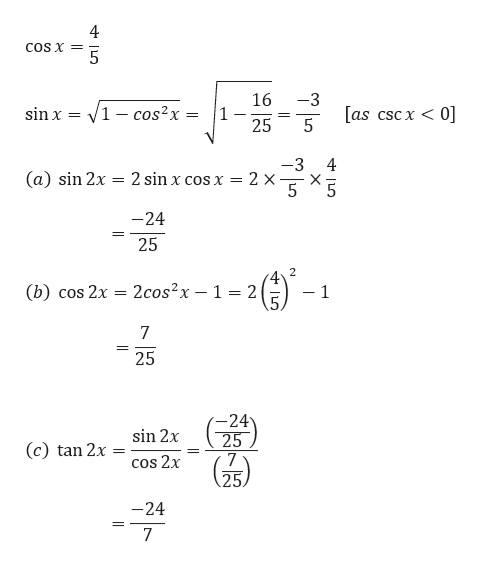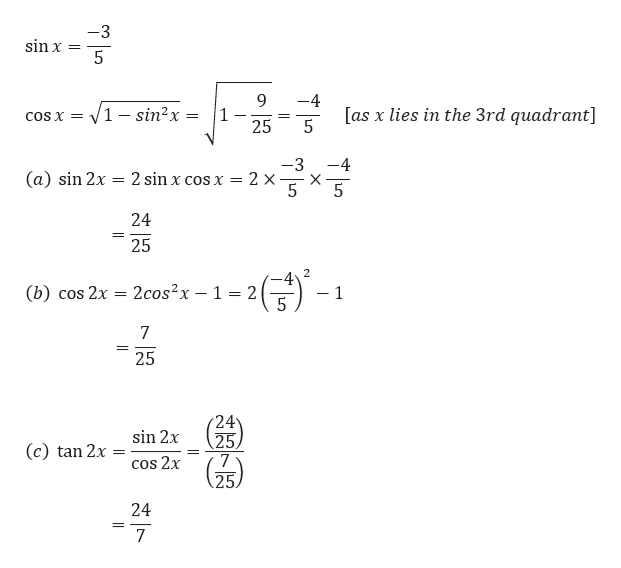# If cosx=4/5,cscx<0,then:sin 2x=_____                  cos 2x=_____            tan 2x=_________f sinx =−3/5, in quadrant III, then:sin 2x=_____ cos 2x=_____ tan 2x=_________If tan x=−1/3,cosx>0,then:sin (2x)=_____       cos (2x)=_____              tan (2x)=_________ Using a double-angle or half-angle formula to simplify the given expressions.(1) If cos2(37∘)−sin2(37∘)=cos(A∘),then:A=___________degrees2) If cos2(7x)−sin2(7x)=cos(B),then:B=___________

Question
44 views

If cosx=4/5,cscx<0,then:

sin 2x=_____                  cos 2x=_____            tan 2x=_________

f sinx =−3/5, in quadrant III, then:

sin 2x=_____ cos 2x=_____ tan 2x=_________

If tan x=−1/3,cosx>0,then:

sin (2x)=_____       cos (2x)=_____              tan (2x)=_________

Using a double-angle or half-angle formula to simplify the given expressions.

(1) If cos2(37∘)−sin2(37∘)=cos(A∘),then:

A=___________degrees

2) If cos2(7x)−sin2(7x)=cos(B),then:

B=___________

check_circle

Step 1

NOTE: As you have posted question with multiple subparts, we will answer the first three questions for you as per our policy. If you need help with the solution of other subparts, kindly repost them separately.

Step 2

Given,help_outlineImage Transcriptionclose4 COS X 5 16 3 as cscx0] 1 - cos2x sin x = = 3 2 sin x cos x = 2 x 4 (a) sin 2x = -24 25 4 2cos2x 1 2 2 (b) cos 2x - 1 7 25 -24 25 sin 2x (c) tan 2x = 7 COs 2x -24 7 LO L LO fullscreen
Step 3

Given,

...help_outlineImage Transcriptionclose-3 sin x = 5 4 V1 - sin2x [as x lies in the 3rd quadrant] 5 1 25 COS X= -3 (a) sin 2x 2 sin x cos x = 2 X X 24 25 -4 2 - 1 2cos2x 1 2 (b) cos 2x 7 25 sin 2x 25 tan 2x COS 2x 25. 24 7 fullscreen

### Want to see the full answer?

See Solution

#### Want to see this answer and more?

Solutions are written by subject experts who are available 24/7. Questions are typically answered within 1 hour.*

See Solution
*Response times may vary by subject and question.
Tagged in

### Trigonometric Ratios